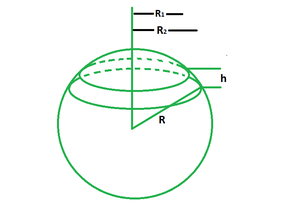Open in App
Not now

# Spherical Segment Formula

• Last Updated : 27 Apr, 2022

A spherical segment is a part of a sphere formed when a plane slices the sphere at the top and bottom in such a way that both cuts are parallel to one another. It corresponds to a spherical frustum because it resembles a spherical cap with the top truncated. A zone is the surface of the spherical segment (excluding the bases).### Spherical Segment Formula

A spherical segment has two major formulae, that is, its area and volume. Below is a detailed explanation of the formula for both area and volume of a spherical segment.

Area of a Spherical Segment

The area of a spherical segment is defined as the area covered by a spherical segment in a three-dimensional space. It is equal to the product of its radius and height with a constant of 2π.

A = 2πRh

Where,

r is the radius of segment,

h is the height of the segment.

Volume of a Spherical Segment

The volume of a spherical segment is defined as the space enclosed within the segment. It is equal to the product of the height and the sum of thrice the square of the first radius, thrice the square of the second radius, and the square of height with a constant of π/6.

V = πh(3R12 + 3R22 + h2)/6

Where,

R1 is the radius of small bounded region,

R2 is the radius of large bounded region,

h is the height of the segment.

### Sample problems

Problem 1: Find the area of a segment of a sphere if the radius of the sphere is 14 cm and the height is 7 cm.

Solution:

We have, R = 14 and h = 7.

Using the formula we have,

A = 2πRh

= 2 (22/7) (14) (7)

= 2 (22) (2) (7)

= 616 sq. cm

Problem 2: Find the height of a segment of a sphere if the radius of the sphere is 14 cm and the area is 176 sq. cm.

Solution:

We have, R = 14 and A = 176.

Using the formula we have,

A = 2πRh

=> 176 = 2 (22/7) (14) (h)

=> 176 = 2 (22) (2) (h)

=> 4h = 8

=> h = 2 cm

Problem 3: Find the volume of a segment of a sphere if the radius of the sphere is 14 cm, the radius of the base is 10 cm, and the height is 7 cm.

Solution:

We have, R1 = 14, R2 = 10 and h = 7.

Using the formula we have,

V = πh(3R12 + 3R22 + h2)/6

= (22/7) (7) (3(14)2 + 3(10)2 + 72)/6

= 22 (588 + 300 + 49)/6

= 20614/6

= 3435.66 cu. cm

Problem 4: Find the volume of a segment of a sphere if the radius of the sphere is 8 cm, the radius of the base is 5 cm and the height is 3 cm.

Solution:

We have, R1 = 8, R2 = 5 and h = 3.

Using the formula we have,

V = πh(3R12 + 3R22 + h2)/6

= (22/7) (3) (3(8)2 + 3(5)2 + 32)/6

= 66 (276)/42

= 18216/42

= 433.71 cu. cm

Problem 5: Find the volume of a segment of a sphere if the radius of the sphere is 7 cm, the radius of the base is 14 cm and the area is 264 sq. cm.

Solution:

We have, R1 = 14, R2 = 7 and A = 264

Using the formula we have,

A = 2πRh

=> 264 = 2 (22/7) (14) (h)

=> 264 = 2 (22) (2) (h)

=> 4h = 12

=> h = 3 cm

So, the volume is,

V = πh(3R12 + 3R22 + h2)/6

= (22/7) (3) (3(14)2 + 3(7)2 + 32)/6

= (22/7) (3) (744)/6

= 49104/42

= 1169.14 cu. cm

Problem 6: Find the area of a segment of a sphere if the radius of the sphere is 20 cm and the height is 10 cm.

Solution:

We have, R = 20 and h = 10.

Using the formula we have,

A = 2πRh

= 2 (22/7) (20) (10)

= 2 (22/7) (200)

= 1257.14 sq. cm

Problem 7: Find the volume of a segment of a sphere if the radius of the sphere is 4 cm, the radius of the base is 2 cm and the height is 6 cm.

Solution:

We have, R1 = 4, R2 = 2 and h = 6.

Using the formula we have,

V = πh(3R12 + 3R22 + h2)/6

= (22/7) (6) (3(4)2 + 3(2)2 + 62)/6

= (22/7) (96)

= 18216/42

= 301.71 cu. cm

My Personal Notes arrow_drop_up